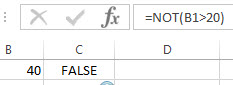# Excel NOT Function

This post will guide you how to use Excel NOT function with syntax and examples in Microsoft excel.

### Description

The Excel NOT function returns the opposite of a given logical or Boolean value. For example, if you supplied with the value TRUE, the NOT function will return FALSE; If you supplied with the value FALSE, and the NOT function will TRUE. So you can use the NOT function to reverse the behavior of an expression or another function.

The NOT function is a build-in function in Microsoft Excel and it is categorized as a Logical Function.

The NOT function is available in Excel 2016, Excel 2013, Excel 2010, Excel 2007, Excel 2003, Excel XP, Excel 2000, Excel 2011 for Mac.

### Syntax

The syntax of the NOT function is as below:

`=NOT(logical)`

Where the NOT function argument is:

• Logical -This is a required argument.  A logical value or expression that can be evaluated as TRUE or FALSE. And the supplied logical argument must be a logical or numeric value.

### Excel NOT Function Examples

The below examples will show you how to use Excel NOT Function to get the opposite to a supplied logical value or expression.

1# =NOT(B1>20)If the Cell B1 value is greater than 20, then this formula will return FALSE. And if the Cell B1 value is smaller than 20, this formula will return TRUE

### Related Functions

• Excel OR function
The Excel OR function used to test multiple conditions and returns TRUE if any of the conditions are TRUE. Otherwise, it will return FALSE. The syntax of the OR function is as below:=OR(logical1, [logical2], …)…
• Excel AND function
The Excel AND function returns TRUE if all of arguments are TRUE, and it returns FALSE if any of arguments are FALSE.The syntax of the AND function is as below:= AND (condition1,[condition2],…) …
• Excel IF function
The Excel IF function perform a logical test to return one value if the condition is TRUE and return another value if the condition is FALSE….

### Related Formulas

• Excel IF formula with NOT logical function
If you want to check several test conditions in an excel formula,then take different actions. You can use NOT function in combination with the AND or OR logical function in excel IF function….